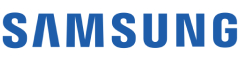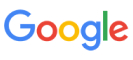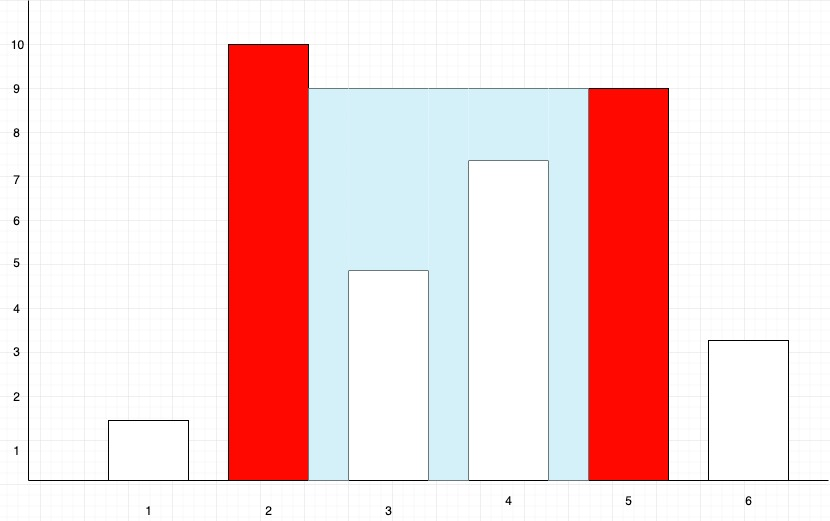New update is available. Click here to update.
Topics

# Container With Most Water

Moderate0/80
Average time to solve is 15m+19 more companies

## Problem statement

Given a sequence of ‘N’ space-separated non-negative integers A,A,A,......A[i]…...A[n]. Where each number of the sequence represents the height of the line drawn at point 'i'. Hence on the cartesian plane, each line is drawn from coordinate ('i',0) to coordinate ('i', 'A[i]'), here ‘i’ ranges from 1 to ‘N’. Find two lines, which, together with the x-axis forms a container, such that the container contains the most area of water.

Note :
``````1. You can not slant the container i.e. the height of the water is equal to the minimum height of the two lines which define the container.

2. Do not print anything, you just need to return the area of the container with maximum water.
``````
Example``````For the above Diagram, the first red marked line is formed between coordinates (2,0) and (2,10), and the second red-marked line is formed between coordinates (5,0) and (5,9). The area of water contained between these two lines is (height* width) = (5-2)* 9 = 27, which is the maximum area contained between any two lines present on the plane. So in this case, we will return 3* 9=27.
``````
Detailed explanation ( Input/output format, Notes, Images )
Constraints:
``````1 <= T <= 50
0 <= N <= 10^4
1 <= A[i] <= 10^5

Time Limit: 1 sec
``````
Sample Input 1 :
``````2
5
4 3 2 1 4
3
1 2 1
``````
Sample Output 1 :
``````16
2
``````
Explanation of The Sample Input 1:
``````For the first case:
We can create ‘n(n+1)/2’ different containers using ‘N' containers for example with 1st and 3rd line we can create a container of area = (3-1)*min(4,2)=4.

All Possible Containers:

Lines used          Area
4,3         area=min(4,3)*1=3
4,2         area=min(4,2)*2=4
4,1         area=min(4,1)*3=3
4,4         area=min(4,4)*4=16
3,2         area=min(3,2)*1=2
3,1         area=min(3,1)*2=2
3,4         area=min(3,4)*3=9
2,1         area=min(2,1)*1=1
2,4         area=min(2,4)*2=4
1,4         area=min(1,4)*1=1

But among all such containers the one with the maximum area will be formed by using the first and last line, the area of which is : (5-1)*min(4,4)=16.

Hence we return 16.

For the second case:
We can take the first and third line to get an area of (3-1)*min(1,1)=2 which is the maximum possible area in this sequence.
``````
Sample Input 2 :
``````2
5
12 4 6 8 1
3
1 2 3
``````
Sample Output 2 :
``````24
2
``````Console